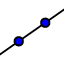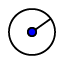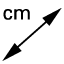# Triangle Congruence? SSA

## Construct two different triangles with a 23° angle that is connected to a side of length 9 but not a side of length 4.

1. Select the SEGMENT WITH GIVEN LENGTHtool, and enter a length of 9. 2. Select the ANGLE WITH GIVEN SIZEtool. Click on B, then A, and then enter 23° as the angle measure. 3. Use the LINEtool to connect A and C. You need to use the line tool because you do not know how long this line will be. 4. Select the CIRCLE: CENTER AND RADIUStool. Click on B and then enter 4 as the length. 5. Use the INTERSECTtool to mark both places where the circle crosses line AC. 6. Use the POLYGONtool to shade in both possible triangles. 7. Use the DISTANCE OR LENGTHtool to measure both triangles. 8. Answer the questions below.

Construct two different triangles with a 23° angle that is connected to a side of length 9 but not a side of length 4. What is the length of the third side of the acute triangle, and what is the length of the third side of the obtuse triangle?

What happens if the 23° angle is connected to the side of length 4 rather than the side of length 9? Give the length of the resulting third side.

Attempt to construct two different triangles with a 23° angle that is connected to a side of length 9 but not a side of length 1. What happens?

Construct a triangle with a 90° angle connected to a side of length 6 but not a side of length 10. What is is length of the third side?

Construct a triangle with a 147° angle connected to a side of length 3 but not a side of length 7. What is is length of the third side?

Summarize what you have learned about using SSA to construct triangles.